## RS Aggarwal Class 7 Solutions Chapter 3 Decimals CCE Test Paper

These Solutions are part of RS Aggarwal Solutions Class 7. Here we have given RS Aggarwal Solutions Class 7 Chapter 3 Decimals CCE Test Paper.

Other Exercises

Question 1.
Solution:
Cost of 1 pen = ₹ 32.50
Cost of 24 such pens = ₹ (32.50 x 24) = ₹ 80
Hence, the cost of 24 pens is ₹ 780.

Question 2.
Solution:
Distance covered by the bus in 1 hour = 64.5 km
Distance covered in 18h = (64.5 x 18) km = 1161 km
Hence, the bus can cover a distance of 1161 km in 18h.

Question 3.
Solution:
First, we will find the product 68 x 65 x 4
Now, 68 x 65 x 4 = 4420 x 4 = 17680
Sum of decimal places in the given decimals = (2 + 1 + 2) = 5
So, the product have five decimal places.
0.68 x 6.5 x 0.04 = 0.17680 = 0.1768

Question 4.
Solution:
Total weight of all the bags = 2231 kg
Weight of each bag = 48.5 kg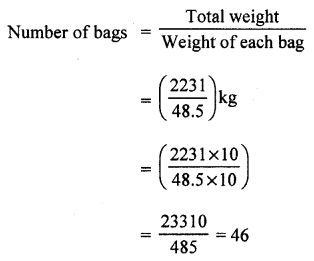Question 5.
Solution: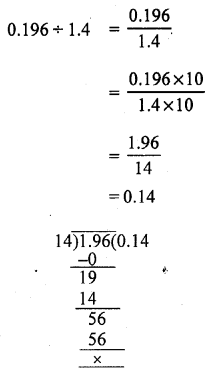Question 6.
Solution:
Product of the given decimals = 1.824
One decimal = 0.64
The other decimal = 1.824 ÷ 0.64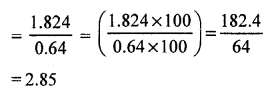Hence, the other decimal is 2.85.

Question 7.
Solution:
Thickness of the pile of plywoods = 2.43 m = 2.43 x 100 cm = 243 cm
Thickness of one piece of plywood = 0.45 cm
Required no. of pieces of plywood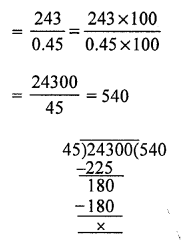Hence, the required number of pieces of plywood is 540.

Question 8.
Solution:
Let the number of sides of the polygon be n.
Length of each side of the polygon = 3.8 cm
Perimeter of the polygon = (3.8 x n) cm
But it is given that its perimeter is 22.8 cm.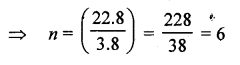Hence, the given polygon has six sides.

Mark (✓) against the correct answer in each of the following :
Question 9.
Solution:
(b) 2.04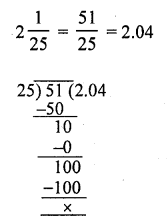Question 10.
Solution:
(b) 1$$\frac { 1 }{ 125 }$$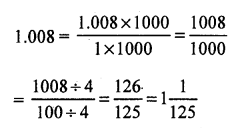Question 11.
Solution:
(c) 2.005 kg
2 kg 5 g = (2 x 1000) g + 5 g = (2005)g
= $$\frac { 2005 }{ 1000 }$$ kg = 2.005 kg

Question 12.
Solution:
(b) 0.08
We have :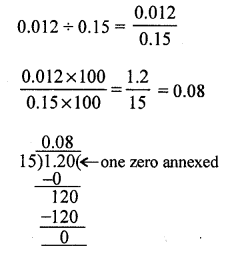Question 13.
Solution:
(c) 0.011
First, we will find the product 11 x 1 x 1
i.e. 11 x 1 x 1 = 11 x 1 = 11
Sum of decimal places in the given decimals = (1 + 1 + 2) = 4
1.1 x 0.1 x 0.01 = 0.0011 [4 places of decimal]

Question 14.
Solution:
(b) 2.03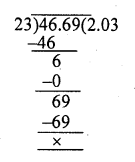Question 15.
Solution:
(c) is correct
Let the number added be x We have :
2.06 + x = 3.1
⇒ x = 3.1 – 2.06
Converting the given decimals into like decimals, we get:
2.06 and 3.10
Thus, required number = (3.10 – 2.06) = 1.04
Hence, 1.04 should be added to 2.06 to get 3.1.

Question 16.
Solution:
(b) 0.06 .
We have :
0.1 – x = 0.04
⇒ x = 0.1 – 0.04
Converting the given decimals into like decimals, we get:
0.10 and 0.04
Thus, required number = (0.10 – 0.04) = 0.06
Hence, 0.06 should be subtracted from 0.1 to get 0.04.

Question 17.
Solution:
(i) 1.001 ÷ 14 = 0.0715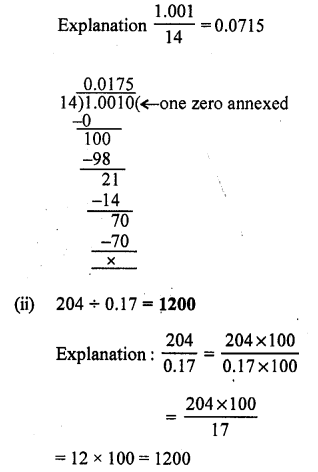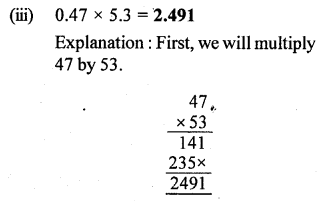47 x 53 = 2491
Sum of decimal places in the given decimals = (2 + 1) = 3
0.47 x 5.3 = 2.491
(iv) 0.023 x 0.03 = 0.69
Explanation first, we will multiply 23 by 3
23 x 3 = 69
Sum of decimal places in the given decimals = (3 + 2) = 5
0.023 x 0.03 =0.00069
(v) (0.7)2 = 0.69
Explanation : (0.7)2 = 0.7 x 0.7
First, we will find the product 0.7 x 0.7
Now, 7 x 7 = 49
Sum of decimal places in the given decimals = (1 + 1) = 2
So, the product must have two decimal places.
(0.7)2 = 0.7 x 0.7 = 0.49
(vi) (0.05)3 = 0.000125
Explanation : First, we will find the
product 0.05 x 0.05 x 0.05
Now, 5 x 5 x 5 = 125
Sum of decimal places in the given decimals = (2 + 2 + 2) = 6
So, the product must have six decimal places.
(0.05)2 = 0.05 x 0.05 x 0.05 = 0.000125

Question 18.
Solution:
(i) False
We have :
0.5 x 0.05 Now, 5 x 5 = 25
Sum of decimal places in the given decimals = (1 + 2) = 3
0.5 x 0.5 = 0.025
(ii) True
We have :
0.25 x 0.8
Now, 25 x 8 = 200
Sum of decimal places in the given decimals = (2 + 1) = 3
0.25 x 0.8 = 0.200 = 0.2
(iii) True
We have :
0.35 ÷ 0.7(iv) False We have :
0.4 x 0.4 x 0.4
Now, 4 x 4 x 4 = 64
Sum of decimal places in the given decimals = (1 + 1 + 1) = 3
0.4 x 0.4 x 0.4 = 0.064
(v) True
6 cm = $$\frac { 6 }{ 100 }$$ m = 0.06 m

Hope given RS Aggarwal Solutions Class 7 Chapter 3 Decimals CCE Test Paper are helpful to complete your math homework.

If you have any doubts, please comment below. Learn Insta try to provide online math tutoring for you.